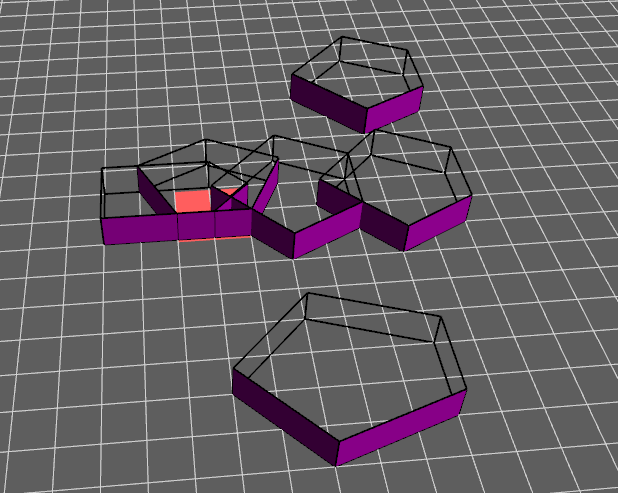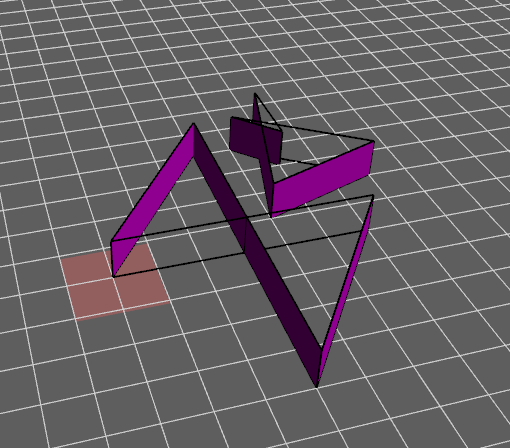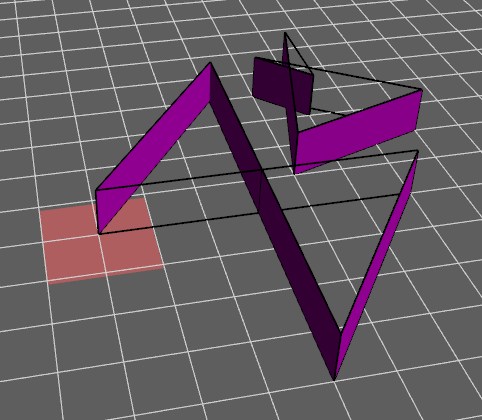### Nikhil Kumar

A stylish blog on my code

Changelog:

# 2D Gilbert-Johnson-Keerthi and Expanding Polytope Algorithm

In this post we will discuss the implementation of GJK (Gilbert-Johnson-Keerthi) and EPA(Expanding Polytope Algorithm) in C++. The projects were created by a team of 3 as part of a class project for Introduction to Computer Graphics at Rutgers University.

## Demonstration

### Example 1### Example 2### Fixed test case of Example 2The output for polygons2.xml shows a collision detected, while observing the polygons there is clearly no collision between the two shapes. This is because the shapes are concave, and our implementation is not able to handle this case. Since the usual solution is to have the engine represent concave shapes as multiple convex shapes for the purposes of collision detection, we can manually modify the testcase to represent the same shapes with more polygons.

The fixed version of polygons2 represents the larger “bow tie” shape as two triangles. The smaller shape is unmodified as the first change was sufficient: the output of this fixed case properly shows that there are no collisions between the two (now three) shapes.

## Gilbert-Johnson-Keerthi Algorithm

GJK is an algorithm which helps us decide if two shapes are colliding. You need to first create a helper method which will calculate the point that will give the maximum dot product in a particular direction.

Next we need a support function which will get us our Minkowski Difference. The Minkowski Difference is the subtraction of every point on the boundary of one shape with every point on the boundary of another shape.

The GJK algorithm checks the Minkowski Difference. If these is an origin in the simplex (a vector full of points), then there is a collision.

The CheckContainsOrigin method checks if the origin is in a triangle formed by 3 points in the simplex The CheckContainsOrigin method is in our github linked on the bottom of this post.

Here is a nice Youtube visualization I found:

## Expanding Polytope Algorithm

The steps to compute EPA are as follows:

1. Get the nearest Edge to the origin
1. Find a support point on that point in the direction of the edge’s normal
1. Get the dot product of the support point and the edge’s normal
1. If the difference between the distance to nearest edge and dot product is less than 0 then we have the penetration vector equal to the normal of the edge and the penetration depth equal to the distance.